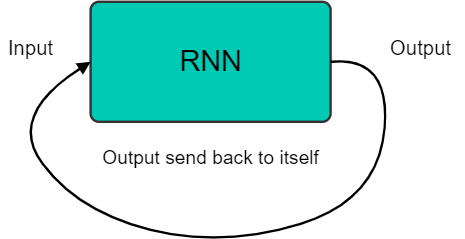## TensorFlow Tutorial Index

The neural network is one of the most well-known machine learning (ML) algorithmic approaches that can outperform other forms of model training in terms of speed and accuracy. The recurrent neural network is one such ML algorithm that uses a sequential approach where we presume that every input and output is independent of other existing layers. This tutorial will give you a crisp idea of what a recurrent neural network (RNN) is and how we can use TensorFlow for the same.

## What Is a Recurrent Neural Network (RNN)?

A Recurrent Neural Network (RNN) is a particular type/class of artificial neural net (ANN) that enables machine learning engineers to model memory units and help persisting data or create prototype short-term dependencies. It follows a sequential/linear fashion where the precise order of the data-points matter. A significant feature of RNN is its hidden state that helps remember different information about the sequence. Another essential factor is that these sequences are usually of arbitrary length. RNN takes the output from the previous step and treats it as input to the current or subsequent step. Here the inputs and outputs are independent of each other. In the case of RNN, the processed output is sent back as input to the algorithm multiple times. It is called time-step, which measures the amount of time the output gets treated as the input for the following sequence of operations.### Real-Life Applications and Implementation of RNN

A recurrent neural network will work similarly to a traditional neural network, except that the memory state will get added to the neurons. Adding computation for including memory is straightforward. RNN also helps in delivering predictive results. Through RNN, developers and ML engineers can perform predictions for various sequential data by offering similar behavior as our human brain does. Companies use Recurrent Neural Network (RNN) algorithms in translation, text generation, text classification, text auto-completion, speech recognition, Named entity recognition, etc. Real-life applications where RNN works implicitly are chatbots, conversational UI, AI-based text to speech converters, searching algorithms, spam detection, video tagging, text summarization, bot detection, and malicious threat identification.

There are numerous advantages of a recurrent neural network. Some of them are listed below:

• Using TensorFlow, we can use RNN to accept input of any length.
• Even when the input size remains significant, it does not impact/increase the model size.
• The design of RNN algorithms is made so that it can remember each piece of information throughout the training phase.
• We can consider RNN as a dynamic neural network that is computationally powerful.
• RNN can easily leverage the internal memory to process any arbitrary series. It does not get performed by other neural networks like feed-forward neural networks.

### Limitations of RNN

There are myriad challenges that recurrent neural network faces because of the characteristics and implementation techniques. Some of them are listed below:

• Due to multiple recurrent execution flows within the algorithm, the entire computation becomes slow.
• It is often difficult to train an RNN model for beginners and even experts.
• If we use activation functions "relu" or "tanh", it becomes problematic and tough to process long-sized sequences within the model.
• RNN is prone to various real-time problems like gradient vanishing and exploding.

### Steps/Algorithm to Train an RNN

1. We have to input a specific dataset or object with data.
2. The network will accept a scenario and perform some random initialized variables.
3. It then computes a predicted result.
4. There will be a difference produced (as an error) while calculating the actual result generated and the expected value.
5. We propagate the model through the same path where the variables get adjusted to identify the error.
6. Repeat steps (1) to (5) until we are confident that the variables for getting the desired output relate to what we are expecting.
7. Such an algorithm creates a systematic prediction by using these variables to obtain further unseen input.